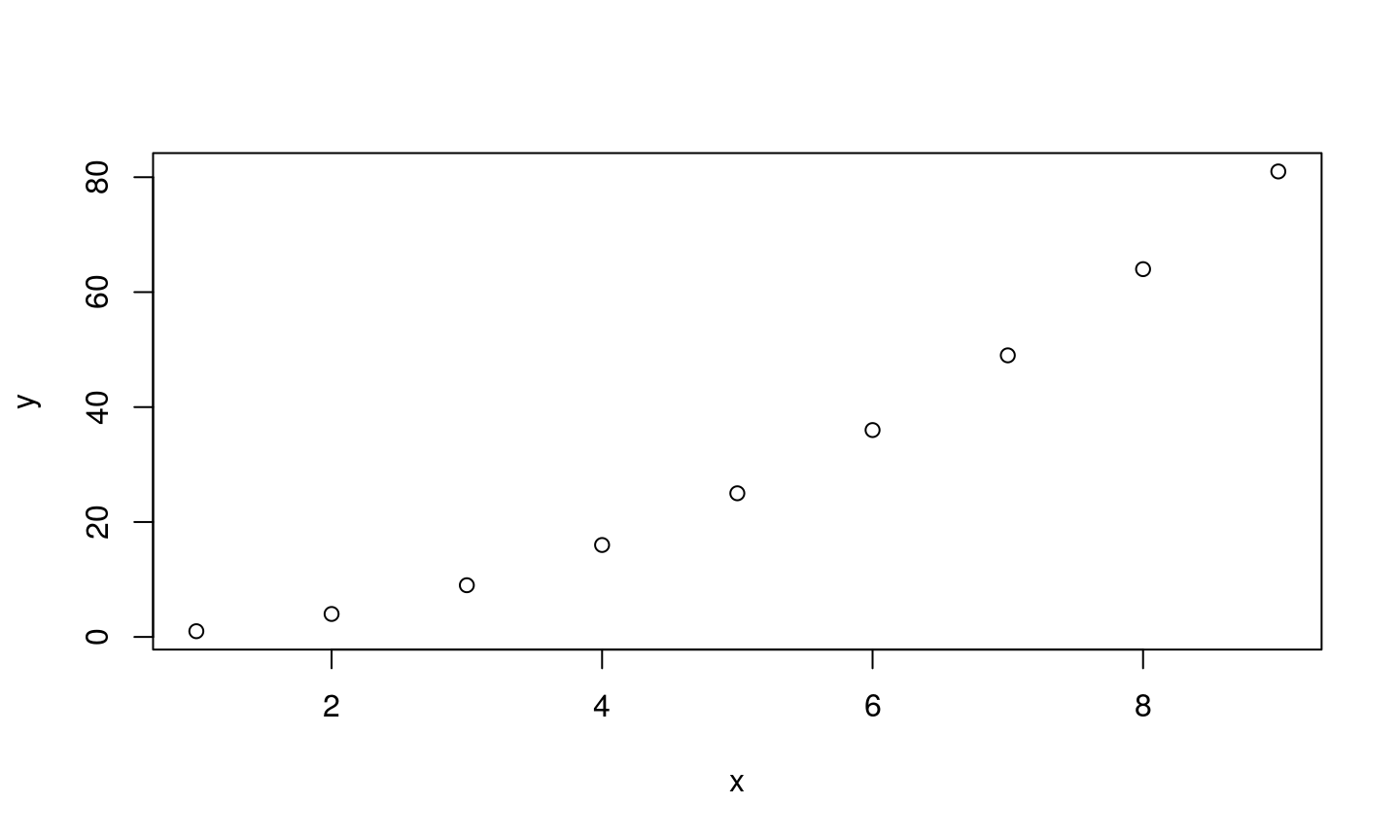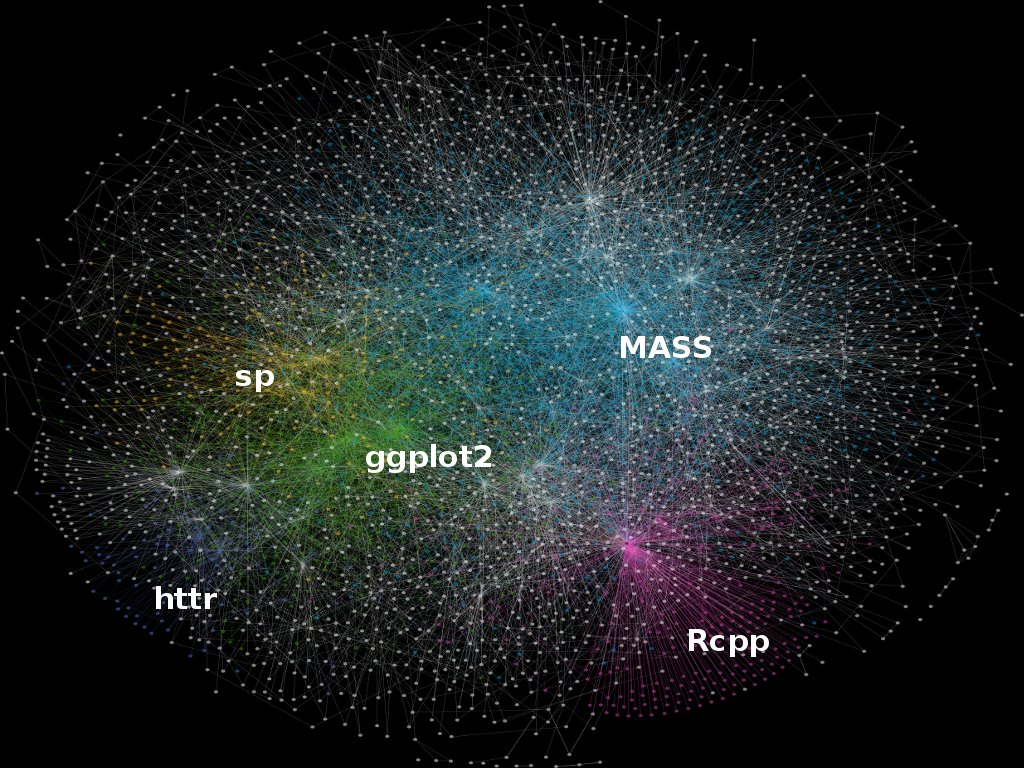Slides: github.com/ITSLeeds/R4TA

An overview of the course

This course teaches two skill-sets that are fundamental in modern transport research: programming and data analytics, with a focus on spatial data. Combining these enables powerful transport planning and analysis workflows for tackling a wide range of problems, including:

• How to effectively handle large transport datasets?
• Where to locate new transport infrastructure?
• How to develop automated and reproducible transport planning workflows?
• How can increasingly available datasets on air quality, traffic and active travel be used to inform policy?
• How to visualise results in an attractive and potentially on-line and interactive manner?

Day 1: Foundations

• Learn about the 'R ecosystem' for transport
• Understand spatial data classes
• Read, process and save a wide variety of datasets
• Spatial data and the tidyverse
• R for air quality data

Day 2: Transport applications

• Working with origin-destination (OD) data
• Converting origin-destination data to lines and routes
• Access and use of OSM data from R
• Route networks
• Visualising transport data

Resources

• The course website/wiki is github.com/ITSLeeds/R4TA
• Geocomputation with R (Lovelace, Nowosad, and Muenchow 2018)
• Chapter 2 on spatial data classes (printed)
• Chapter 7 on transport applications (printed)
• Chapters 3 + 6 on the tidyverse + data I/O
• Efficient R Programming (Gillespie and Lovelace 2016)
• stplanr: A package for Transport Planning (stplanr-paper)

Features

Source: https://www.r-bloggers.com/on-the-growth-of-cran-packages/

R is an extremely flexible language. Huge package ecosystem.Scalability

As datasets have grown, so has the importance of efficient computing.

• R was designed for efficiency - lots of 'leg-work' done in C
• R is conducive to cloud and parallel computing
• Easy to parallelise

Visualisation

• Vital for communication
• Increasingly interactiveR vs Python"R" > "Python" # ?
##  TRUE

R as a giant calculator

R has a unique syntax (R Core Team 2017)

5 * 5
1 + 4 * 5
4 * 5 ^ 2

Functions and objects

In R:

• Everything that exists is an object
• Everything that happens is a function

E.g., load a data object and find its dimension:

data(mpg, package = "ggplot2") # load the object mpg
dim(mpg) # use a function (dim) to do something with it
##  234  11

Objects

• R is object-orientated
• Objects persist in memory for the duration of the session
• Objects are usually assigned by the <- or = operator (usually identical)
• Any names can be give to R objects, except special characters like \
• Exercise: what do these commands do?
a = 1
b = 2
c = "c"
x_thingy = 4
a + b
a * b
a + c
a / x_thingy

ls()
##  "a"        "b"        "c"        "mpg"      "x_thingy"
x = x_thingy
rm(x_thingy)
x
##  4
ls()
##  "a"   "b"   "c"   "mpg" "x"

Functions

"R, at its heart, is a functional programming (FP) language. This means that it provides many tools for the creation and manipulation of functions."

• All functions have brackets (to run)
• The arguments of the functions go in the brackets
replicate(n = 3, expr = x)
##  4 4 4
exp(x)
##  54.59815

Basic plotting

x = 1:9
y = x^2
plot(x = x, y =y)Demonstration then exercise: Getting used to RStudio and R

• Open RStudio and have a look around
• Create a new project
• Create a new R Script: pass code to the console with Ctl-Enter
• Use R as a calculator: what is:
• Explore each of the 'panes'
• Find and write down some useful shortcuts (Alt-Shift-K on Windows/Linux)

Printing the result during assignment

# Assignment of x
x = 5
x
##  5
# A trick to print x
(x = 5)

The console or the script pane?

• Is it part of a longer story that will ultimately be shared? (use script files)
• Is it just playing that does not need to be stored? (use the console)

You can switch effortlessly between them with Ctl+1 and Ctl+2.

Other shortcuts:

• Magic Tab button
• Pressing Up in console
• See Alt-Shift-K for more

Assignment

• Be warned: easy to overwrite data
x = 5 # the same as x <- 5
(x = x + 1)
##  6
• Warning when using =
system.time({x_big = (1:1e7)^2})
system.time({x_big = 1:1e7})
• Warning when using <-
x < -5
x <-5
library(tidyverse)
5 %>%
sin(.) %>%
cos(.) -> res
res = cos(sin(5))

The R ecosystemChoosing packages

• With so many packages, simply choosing the right package can be hard work!
• Fortunately there are well-known ways to decide on which package to use (see section 4.4 of Efficient R Programming):
• Is it mature?
• Is it actively developed?
• Is it well documented?
• Is it well used?